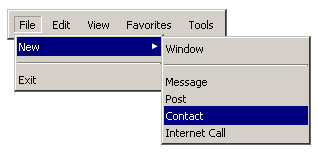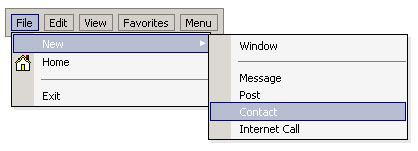Posts Tagged ‘ javascript ’

几种网页转址与“301 永久搬移”的语法

• 302: 暂时搬移 (Temporarily Moved)
• 301: 永久搬移 (Permanently Moved)

doiMenu - 简单好用的 DHTML 选单程式 *

doiMenu 是一支简单好用的 DHTML 选单程式，目前的版本是 1.5。Flash Bar Code 1.0 - Flash 条码产生器 *

• 名　　称: Flash Bar Code
• 版　　本: 1.0
• 档案大小: 18 KB
• 版本性质: Freeware
• 原创网站: AEU-Studio
• 档案下载:

Everything is Object in JavaScript

var ary = [123, "abc"];

Create Objects in JavaScript

var main = new Object;     // 建立新物件
main.x = 123; // 设定物件成员变量(属性)之一
main["y"] = "XYZ"; // 设定物件成员变量(属性)之二

List All Members in an Object

function listMember(main) {
var s = "";
for( key in main )  // 使用 in 运算子列举所有成员
s += key + ": " + main[key] + "\n";
return s;
}

var ary = [123, "abc"];

Construct Object with Initial Value

function Array2DVar(x,y) {  // 定义二维阵列原型
this.length = x;
this.x = x;  // x 维度长度
this.y = y;  // y 维度长度
for(var i = 0; i < this.length; i++)  // 初始各元素值为 null
this[i] = new Array(y);  // this 代表物件本身
}

var a2dv = new Array2DVar(10, 10);  // 建立新的 10*10 的二维阵列
a2dv = "ABC";  // 设定二维阵列元素值
a2dv = "XYZ";
a2dv = 1000;

alert( a2dv);  // 取得二维阵列元素值，并显示出来

Initial Array Object

a = new Array("abc", "xyz", 1000); // constructor 语法，或
a = ["abc", "xyz", 1000]; // 阵列标准语法

b = [ // 使用函式作为阵列元素
function () { alert("这个好玩!") },
function () { alert("再按一次离开!") },
function () { alert("再来一次!") },
function () { alert("最后一次!") }
];

for (var i = 0; i < b.length ; i++)
b[i]();

Object as Association Array

obj1 = {"a" : "Athens" , "b" : "Belgrade", "c" : "Cairo"};
alert(obj1["a"]);  // 显示 Athens
obj2 = {
name: "Edward",
showName: function() { alert(this.name); }  // 使用函式作为物件属性
}
obj2.showName();  // 显示 Edward
obj2.age = 23;  // 属性可以动态加入

Object as Return Value

function a () {
return [32, 17];
}
b = a();
alert( b ); // 或
function pixel () {
return {"x": 32, "y":17};
}
point = pixel ();
alert (point.x + "\n" + point.y);  // 或
alert (pixel().x + "\n" + pixel().y);

Delegation Function Object

function doloop(begin, end, func) {  // 这个函式是个 iterator
for (var i = begin; i < end; i++) {
func(i);
}
}
function func1(i) {  // 印出 ** n **
document.writeln("** " + i + " **<br>");
}
doloop(1, 10, func1);  // 印出 10 行 ** n **
doloop(20, 29,
function(i) {  // 印出 10 行 ## n ##
document.writeln("## " + i + " ##<br>");
}
);

Object = Properties + Behaviors

function Array2D(x,y){  // 以一维阵列模拟二维阵列的原型物件
this.length = x * y;  // 阵列总长
this.x = x;  // x 维度长度
this.y = y;  // y 维度长度
for(var i = 0; i < this.length; i++)  // 初始各元素值为 null
this[i] = null;
this.get = function(x,y){  // 成员函式：取得阵列第 [x,y]个元素值
return this[x*this.x + y];
}
this.set = function(x,y,value){  // 成员函式：设定阵列第 [x,y] 个元素值
this[x*this.x + y] = value;
}
}

var a2d = new Array2D(10, 10);  // 建立新的“二维”阵列

a2d.set(1, 3, "ABC");  // 设定“二维”阵列元素值
a2d.set(2, 6, "XYZ");
a2d.set(9, 9, 1000);

alert( a2d.get(1,3) );  // 取得“二维”阵列元素值，并显示出来

Member Function Outside of Constructor

function Array2D(x,y){  // 以一维阵列模拟二维阵列的原型物件
this.length = x * y;  // 阵列总长
this.x = x;  // x 维度长度
this.y = y;  // y 维度长度
for(var i = 0; i < this.length; i++)  // 初始各元素值为 null
this[i] = null;
this.get = Array2DGet;  // 用这种方式把成员函式挂进来
this.set = Array2DSet;
}

function Array2DGet(x,y){  // 成员函式：取得阵列第 [x,y] 个元素值
return this[x*this.x + y];
}

function Array2DSet(x,y,value){  // 成员函式：设定阵列第 [x,y] 个元素值
this[x*this.x + y] = value;
}

Dynamic Object Function

function array_max(){  // 定义求取 Array 最大值之函式
var i, max = this;
for (i = 1; i < this.length; i++){
if (max < this[i])
max = this[i];
}
return max;
}
Array.prototype.max = array_max;  // 在 Array 原型中加入 max 函式

var x = new Array(1, 2, 3, 4, 5, 6);  // 透过 Array 建构子建立一阵列，想求取 x 中某一元素之最大值
var y = x.max( );  // 取得 x 之最大元素

Dynamic Mix in

function main(){  // main 之建构子
// ...
this.imports =
function (object) {
if( typeof object == "object")
for( value in object )
this[value] = object[value];
}
// ...
}
obj = new main();
main.imports(new dynamic());  // 汇入 dynamic 物件之所有功能

[转载]Unicode 脱逸序列转换

<META HTTP-EQUIV="Content-Type" CONTENT="text/html; charset=big5">
<script language=javascript>
<!--
function StringToUniCode(source) {
sret = "";
// Unicode 跳脱序列之格式为 \u00ff
for(var i=0; i < source.length; i++) {
var num = new Number( source.charCodeAt(i));
sret += "\\u" + num.toString(16);
}
return sret;
}
function convert(){
document.forms.content.result.value = StringToUniCode(document.forms.content.source.value);
}
//-->
</script>

<form name=content>

<input type=button value=转换 onclick="convert()">
</form>

HTTP 的协议本身是“无记忆状态 (Stateless) ”的，无论是用户端还是 Web 服务器，每一个页面都没有关联，每一次的请求都是单独的请求。服务器借由 Cookie 来让同一个用户的各项操作都可以产生关联。

IE 浏览器从“工具”选单 -> Intertnet 选项 -> 安全性，按“自订层级”，到 Cookie 的部分，全都设为关闭，按确定，关闭浏览器，再重新启动浏览器即可。

IE 的 Cookie 默认存放位置是在：C:\WINDOWS\Temporary Internet Files

什么是 Session?

Session 的资料是存放在 Web 服务器端。用解释的不好解释，我们先来举个例子...